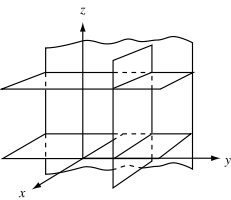Search IntMath
Close

# Cartesian Form in Geometry## Introduction

Cartesian form is a method of representing points on a Euclidean plane using coordinates. It is also known as coordinate geometry or graphing. It is a powerful tool in mathematics that allows us to graph linear equations and to visualize relationships between points and lines. Cartesian form can be used to solve a variety of problems, from basic geometry to more complicated algebraic equations.

## Coordinates

A coordinate is a pair of numbers that describe the position of a point on a coordinate plane. The first number is the x-coordinate, which represents the point's position along the horizontal axis. The second number is the y-coordinate, which represents the point's position along the vertical axis. The origin of the coordinate plane is the point (0, 0).

For example, the point (3, 4) would be located 3 units to the right of the origin on the x-axis, and 4 units up on the y-axis. We can use coordinates to graph points on a coordinate plane and to solve equations.

## Graphing

Once we have the coordinates of a point, we can graph it on a coordinate plane. To do this, we simply plot the point using the x and y coordinates. We can also graph linear equations by plotting two points and connecting them with a line. This allows us to visualize the equation in a more intuitive way.

For example, let's say we have the equation y = 2x + 1. We can graph this equation by plotting two points: (0, 1) and (1, 3). Then, we can connect the two points with a line. This will create a line that has a slope of 2 and a y-intercept of 1.

## Solving Equations

Once we have graphed an equation, we can use the visual representation to solve for the unknown variables. For example, if we have the equation y = 2x + 1, we can use the graph to find the value of x when y = 4. We can do this by finding the point (x, 4) on the graph, which will be (2, 4). This means that x = 2 when y = 4.

We can also use the graph to solve more complicated equations. For example, if we have the equation y = x2 + 3x + 2, we can use the graph to find the roots of the equation. The roots are the x-values where the line crosses the x-axis. By finding these points on the graph, we can calculate the roots of the equation.

## Practice Problems

1. Graph the equation y = 3x - 2.

Answer: We can graph this equation by plotting two points: (0, -2) and (1, 1). Then, we can connect the two points with a line. This will create a line that has a slope of 3 and a y-intercept of -2.

2. Solve the equation y = x2 + 3x + 2 for x when y = -1.

Answer: We can solve this equation by graphing the equation on a coordinate plane. The point (x, -1) will be (-2, -1). This means that x = -2 when y = -1.

3. Find the y-intercept of the equation y = -4x + 6.

Answer: The y-intercept of this equation is 6. This is because the y-intercept is the point where the line crosses the y-axis. In this equation, the y-intercept is 6 because when x = 0, y = 6.

4. Graph the equation y = x2 - 5.

Answer: We can graph this equation by plotting two points: (0, -5) and (1, -4). Then, we can connect the two points with a line. This will create a parabola that has a y-intercept of -5.

5. Solve the equation y = 4x - 2 for x when y = 8.

Answer: We can solve this equation by graphing the equation on a coordinate plane. The point (x, 8) will be (2, 8). This means that x = 2 when y = 8.

6. Find the roots of the equation y = 2x2 + 4x - 6.

Answer: We can find the roots of this equation by graphing the equation on a coordinate plane. The roots are the x-values where the line crosses the x-axis. In this equation, the roots are -3 and 1.

## Summary

Cartesian form is a powerful tool in mathematics that allows us to graph linear equations and visualize relationships between points and lines. We can use coordinates to graph points on a coordinate plane and to solve equations. Graphing equations allows us to visualize the relationships between variables and to solve for unknowns. We can also use the graph to find the roots of an equation.

## FAQ

### What is a Cartesian Form?

A Cartesian form is a form of mathematical representation that uses a pair of numbers to indicate the position of a point on a graph. The point is located by the intersections of two perpendicular lines, usually referred to as the x-axis and the y-axis. The coordinates of the point are then expressed as an ordered pair (x, y).

### How is the Cartesian Form Used?

The Cartesian form is used to graph equations and to calculate the distance between two points. It is also used to find the slope of a line and the equation of a line.

## Problem SolverThis tool combines the power of mathematical computation engine that excels at solving mathematical formulas with the power of GPT large language models to parse and generate natural language. This creates math problem solver thats more accurate than ChatGPT, more flexible than a calculator, and faster answers than a human tutor. Learn More.